# GRE Math : How to subtract rational expressions with different denominators

## Example Questions

### Example Question #5 : Rational Expressions

Choose the answer which best simplifies the following expression: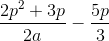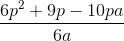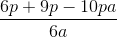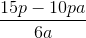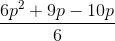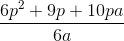Explanation:

To simplify this expression, first multiply both terms by the denominator of the other over itself: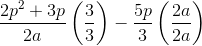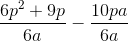Now that you have a common denominator, you may subtract:### Example Question #1 : How To Subtract Rational Expressions With Different Denominators

Choose the answer which best simplifies the following expression: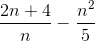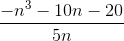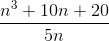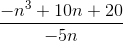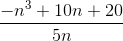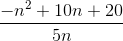Explanation:

To simplify this problem, multiply each term by the denominator of the other over itself: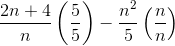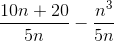Now that both terms share a denominator, you can subtract:### Example Question #2 : How To Subtract Rational Expressions With Different Denominators

Choose the answer which best simplifies the following expression: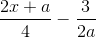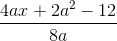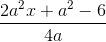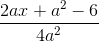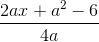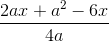Explanation:

To solve this problem, first multiply each term of the original expression by the denomenator of the other over itself: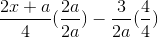Then you will have two terms with a common denomenator: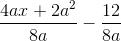### Example Question #3 : How To Subtract Rational Expressions With Different Denominators

Choose the answer which best simplifies the expression below: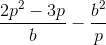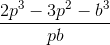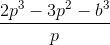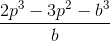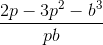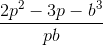Explanation:

To simplify this problem, multiply each term by the denomenator of the other over itself: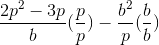Then you will yield terms with a like denomenator, which can be combined: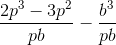### Example Question #4 : How To Subtract Rational Expressions With Different Denominators

Choose the answer which best simplifies the expression below: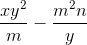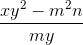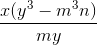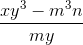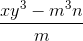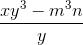Explanation:

To simplify the expression, first multiply each term by the denomenator of the other over itself: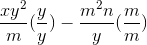Then you yield terms with common denomenators, which can be combined: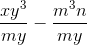### Example Question #5 : How To Subtract Rational Expressions With Different Denominators

Choose the answer which best simplifies the following expression: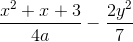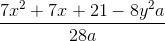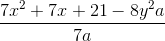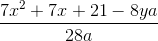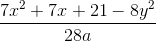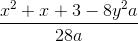Explanation:

To simplify, first multiply each of the terms by the denomenator of the other over itself: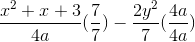Then you will get terms with a common denomenator, which can be combined: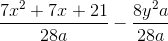### Example Question #6 : How To Subtract Rational Expressions With Different Denominators

Choose the answer which best simplifies the expression below: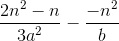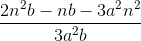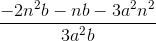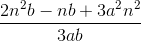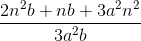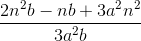Explanation:

To simplify, first multiply each of the terms by the denomenator of the other over itself: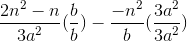You will yield terms with a common denomenator, which can be combined: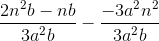### Example Question #7 : How To Subtract Rational Expressions With Different Denominators

Choose the answer which best simplifies the expression below: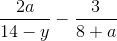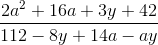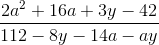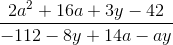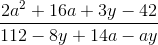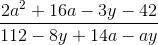Explanation:

To simplify, multiply each of the terms by the denomenator of the other, over itself: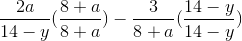You will yield terms with a common denomenator, which can be combined: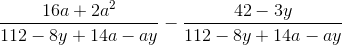Tired of practice problems?

Try live online GRE prep today.